Caribou
caribou::geometry::Element< Derived, ScalarType > Struct Template Reference

## Classes

struct  GaussNode

## Public Types

using Scalar = ScalarType

template<INTEGER_TYPE Dim>
using Vector = Eigen::Matrix< Scalar, Dim, 1 >

template<INTEGER_TYPE Rows, INTEGER_TYPE Cols, int Options = Eigen::ColMajor>
using Matrix = Eigen::Matrix< Scalar, Rows, Cols, Options >

template<INTEGER_TYPE Rows, INTEGER_TYPE Cols, int Options = Eigen::ColMajor>
using MatrixI = Eigen::Matrix< Scalar, Rows, Cols, Options >

using LocalCoordinates = Vector< CanonicalDimension >

using WorldCoordinates = Vector< Dimension >

## Public Member Functions

auto number_of_nodes () const -> UNSIGNED_INTEGER_TYPE
Get the number of nodes in the element.

auto number_of_gauss_nodes () const -> UNSIGNED_INTEGER_TYPE
Get the number of gauss nodes in the element.

auto node (const UNSIGNED_INTEGER_TYPE &index) const
Get the Node at given index.

auto nodes () const
Get the set of nodes.

auto gauss_node (const UNSIGNED_INTEGER_TYPE &index) const -> const GaussNode &
Get the gauss node at given index.

auto gauss_nodes () const -> const std::vector< GaussNode > &
Get the set of gauss nodes.

auto number_of_boundary_elements () const
Get the number of boundary elements (ex. More...

auto boundary_elements_node_indices () const -> const auto &
Get the list of node indices of the boundary elements. More...

auto boundary_element (const UNSIGNED_INTEGER_TYPE &boundary_id) const
Construct and return the given boundary element. More...

auto L (const LocalCoordinates &xi) const -> Vector< NumberOfNodesAtCompileTime >
Get the Lagrange polynomial values evaluated at local coordinates xi w.r.t each element's interpolation nodes. More...

auto dL (const LocalCoordinates &xi) const -> Matrix< NumberOfNodesAtCompileTime, CanonicalDimension >
Get the Lagrange polynomial derivatives w.r.t the local frame {dL/du} evaluated at local coordinates {u} w.r.t each segment's interpolation nodes. More...

auto center () const -> WorldCoordinates
Get the position at the center of the element.

auto world_coordinates (const LocalCoordinates &coordinates) const -> WorldCoordinates
Get the world coordinates of a point from its local coordinates.

auto local_coordinates (const WorldCoordinates &coordinates) const -> LocalCoordinates
Get the local coordinates of a point from its world coordinates by doing a set of Newton-Raphson iterations. More...

auto local_coordinates (const WorldCoordinates &coordinates, const LocalCoordinates &starting_point, const FLOATING_POINT_TYPE &residual_tolerance, const UNSIGNED_INTEGER_TYPE &maximum_number_of_iterations) const -> LocalCoordinates
Get the local coordinates of a point from its world coordinates by doing a set of Newton-Raphson iterations. More...

auto contains_local (const LocalCoordinates &xi, const FLOATING_POINT_TYPE &eps=1e-10) const -> bool
Return true if the element contains the point located at the given local coordinates. More...

template<typename MatrixType >
auto interpolate (const LocalCoordinates &coordinates, const Eigen::MatrixBase< MatrixType > &values) const
Interpolate a value at local coordinates from the given interpolation node values. More...

auto jacobian (const LocalCoordinates &coordinates) const -> Matrix< Dimension, CanonicalDimension >
Compute the Jacobian matrix of the transformation T(xi)-> x evaluated at local coordinates xi. More...

## Static Public Attributes

static constexpr auto CanonicalDimension = traits<Derived>::CanonicalDimension

static constexpr auto Dimension = traits<Derived>::Dimension

static constexpr auto NumberOfNodesAtCompileTime = traits<Derived>::NumberOfNodesAtCompileTime

static constexpr auto NumberOfGaussNodesAtCompileTime = traits<Derived>::NumberOfGaussNodesAtCompileTime

## ◆ caribou::geometry::Element::GaussNode

 struct caribou::geometry::Element::GaussNode
Class Members
LocalCoordinates position
Scalar weight

## ◆ boundary_element()

template<typename Derived , typename ScalarType = FLOATING_POINT_TYPE>
 auto caribou::geometry::Element< Derived, ScalarType >::boundary_element ( const UNSIGNED_INTEGER_TYPE & boundary_id ) const
inline

Construct and return the given boundary element.

Example:

Hexahedron<Linear> hexa;

## ◆ boundary_elements_node_indices()

template<typename Derived , typename ScalarType = FLOATING_POINT_TYPE>
 auto caribou::geometry::Element< Derived, ScalarType >::boundary_elements_node_indices ( ) const -> const auto &
inline

Get the list of node indices of the boundary elements.

The return type is up to the implementation but should normally be:

1. Dynamic number of boundary element where each boundary element has a dynamic number of nodes std::vector<std::vector<unsigned int>>
2. Dynamic number of boundary element where each boundary element has a static number of nodes std::vector<std::array<unsigned int, traits<BoundaryElement>::NumberOfNodesAtCompileTime>>
3. Static number of boundary element where each boundary element has a dynamic number of nodes std::array<std::vector<unsigned int>, traits<Element>::NumberOfBoundaryElementsAtCompileTime>
4. Static number of boundary element where each boundary element has a static number of nodes std::array< std::array<unsigned int, traits<BoundaryElement>::NumberOfNodesAtCompileTime>, traits<Element>::NumberOfBoundaryElementsAtCompileTime

## ◆ contains_local()

template<typename Derived , typename ScalarType = FLOATING_POINT_TYPE>
 auto caribou::geometry::Element< Derived, ScalarType >::contains_local ( const LocalCoordinates & xi, const FLOATING_POINT_TYPE & eps = 1e-10 ) const -> bool
inline

Return true if the element contains the point located at the given local coordinates.

Parameters
 coordinates Local coordinates of a point eps If the given point is located barely outside the element, which is, less than this eps value, returns true.

## ◆ dL()

template<typename Derived , typename ScalarType = FLOATING_POINT_TYPE>
 auto caribou::geometry::Element< Derived, ScalarType >::dL ( const LocalCoordinates & xi ) const -> Matrix
inline

Get the Lagrange polynomial derivatives w.r.t the local frame {dL/du} evaluated at local coordinates {u} w.r.t each segment's interpolation nodes.

Example:

// Computes the derivatives of node #2 Lagrange polynomial evaluated at local coordinates {-0.4}
float dp = Segment2::dL(-0.4);

## ◆ interpolate()

template<typename Derived , typename ScalarType = FLOATING_POINT_TYPE>
template<typename MatrixType >
 auto caribou::geometry::Element< Derived, ScalarType >::interpolate ( const LocalCoordinates & coordinates, const Eigen::MatrixBase< MatrixType > & values ) const
inline

Interpolate a value at local coordinates from the given interpolation node values.

The values at nodes must be provided in an Eigen::Matrix where the row i of the matrix is the value (as a row-vector or a scalar) at the node i.

Template Parameters
 MatrixType Type of the Eigen matrix containing the values at nodes.

## ◆ jacobian()

template<typename Derived , typename ScalarType = FLOATING_POINT_TYPE>
 auto caribou::geometry::Element< Derived, ScalarType >::jacobian ( const LocalCoordinates & coordinates ) const -> Matrix
inline

Compute the Jacobian matrix of the transformation T(xi)-> x evaluated at local coordinates xi.

The Jacobian is defined as:

*
* |dx|     |du|
* |dy| = J |dv|
* |dz|     |dw|
*
* 1D canonical element
* --------------------
*
* 1D manifold:    J(u) = dx/du = sum_i dNi/du * x_i
*                 det(J) = |J|
*
* 2D manifold:    J(u)  = | dx/du | = | sum dNi/du x_i |
*                         | dy/du | = | sum dNi/du y_i |
*                 det(J) = sqrt(J.dot(J))
*
*                        | dx/du | = | sum dNi/du x_i |
* 3D manifold:    J(u) = | dy/du | = | sum dNi/du y_i |
*                        | dz/du | = | sum dNi/du z_i |
*                 det(J) = sqrt(J.dot(J))
*
*
* 2D canonical element
* --------------------
*
* 1D manifold:    N/A
*
* 2D manifold:    J(u,v) = | dx/du   dx/dv |   | sum dNi/du  x_i    sum dNi/dv  x_i |
*                          | dy/du   dy/dv | = | sum dNi/du  y_i    sum dNi/dv  y_i |
*                 det(J) = |det(J)|
*
*                          | dx/du   dx/dv |   | sum dNi/du  x_i    sum dNi/dv  x_i |
* 3D manifold:    J(u,v) = | dy/du   dy/dv | = | sum dNi/du  y_i    sum dNi/dv  y_i |
*                          | dz/du   dz/dv | = | sum dNi/du  z_i    sum dNi/dv  z_i |
*                 det(J) = sqrt((J.transpose() * J).determinant())
*                        = J.col(0).cross(J.col(1)).norm();
*
*
* 3D canonical element
* --------------------
*
* 1D manifold:    N/A
* 2D manifold:    N/A
*                            | dx/du   dx/dv   dx/dw |   | sum dNi/du x_i   sum dNi/dv x_i    sum dNi/dw x_i |
* 3D manifold:    J(u,v,w) = | dy/du   dy/dv   dy/dw | = | sum dNi/du y_i   sum dNi/dv y_i    sum dNi/dw y_i |
*                            | dz/du   dz/dv   dz/dw |   | sum dNi/du z_i   sum dNi/dv z_i    sum dNi/dw z_i |
*
*
*
*
* where dNi/du (resp. dv and dw) is the partial derivative of the shape function at node i
* w.r.t the local frame of the canonical element evaluated at local coordinate  {u, v, w} and
* where {xi, yi and zi} are the world coordinates of the position of node i on its element manifold.
*

Example:

// Computes the Jacobian of a 3D segment and its determinant evaluated at local coordinates 0.5 (half-way through the segment)
Segment<3, Linear> segment {{5, 5, 5}, {10, 5,0}};
Matrix<3,1> J = segment.jacobian (0.5);
double detJ = sqrt(J.dot(J));
// Computes the Jacobian of a 3D triangle and its determinant evaluated at local coordinates {1/3, 1/3} (on its center point)
Triangle<3, Linear> triangle {{5,5,5}, {15, 5, 5}, {10, 10, 10}};
Matrix<3,2> J = triangle.jacobian(1/3., 1/3.);
double detJ = (J^T * J).determinant() ^ 1/2;
// Computes the Jacobian of a 3D tetrahedron and its determinant evaluated at local coordinates {1/3, 1/3, 1/3} (on its center point)
Tetrahedron<3> tetra {{5,5,5}, {15, 5, 5}, {10, 10, 10}};
Matrix<3,3> J = tetra.jacobian(1/3., 1/3., 1/3.);
double detJ = J.determinant();

## ◆ L()

template<typename Derived , typename ScalarType = FLOATING_POINT_TYPE>
 auto caribou::geometry::Element< Derived, ScalarType >::L ( const LocalCoordinates & xi ) const -> Vector
inline

Get the Lagrange polynomial values evaluated at local coordinates xi w.r.t each element's interpolation nodes.

Example:

// Computes the value of node #2 Lagrange polynomial evaluated at local coordinates {-0.4} on a segment
Segment<3, Linear> segment;
float p = segment.L(-0.4);

## ◆ local_coordinates() [1/2]

template<typename Derived , typename ScalarType = FLOATING_POINT_TYPE>
 auto caribou::geometry::Element< Derived, ScalarType >::local_coordinates ( const WorldCoordinates & coordinates ) const -> LocalCoordinates
inline

Get the local coordinates of a point from its world coordinates by doing a set of Newton-Raphson iterations.

local_coordinates() for more details.
Note
By default, the Newton-Raphson will start by an approximation of the local coordinates at [0, 0, 0]. The iterations will stop at 5 iterations, or if the norm o* f relative residual |R|/|R0| is less than 1e-5.

## ◆ local_coordinates() [2/2]

template<typename Derived , typename ScalarType = FLOATING_POINT_TYPE>
 auto caribou::geometry::Element< Derived, ScalarType >::local_coordinates ( const WorldCoordinates & coordinates, const LocalCoordinates & starting_point, const FLOATING_POINT_TYPE & residual_tolerance, const UNSIGNED_INTEGER_TYPE & maximum_number_of_iterations ) const -> LocalCoordinates
inline

Get the local coordinates of a point from its world coordinates by doing a set of Newton-Raphson iterations.

By taking the Taylor expansion of the transformation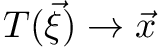with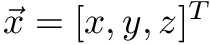being the world coordinates of a point and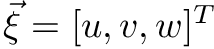its local coordinates within the element, we have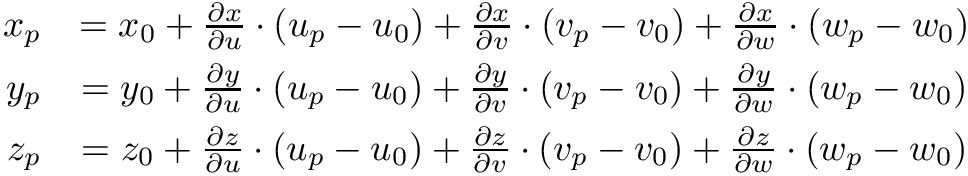where partial derivatives are evaluated at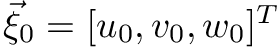. We can reformulate with the following matrix form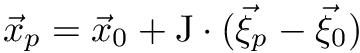where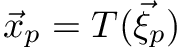,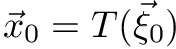and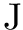is the Jacobian of the transformation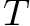. Since we are trying to find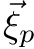, we can rearange the last equation to get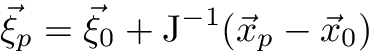Hence, starting from an initial guess at local coordinates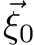, we have the following iterative method: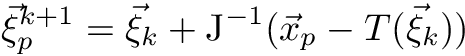The iterations stop when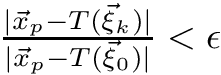Note
When trying to find the local coordinates of non-matching manifolds (for example, the local coordinates of a triangle in a 3D manifold), the following recursive formulae is used: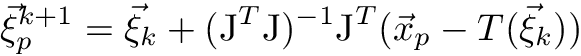Parameters
 coordinates The world coordinates of the point from which we want to get the local coordinates. starting_point An approximation of the real local coordinates we want the get. The closer it is to the solution, the faster the Newton will converge. residual_tolerance The threshold of relative norm of the residual at which point the Newton is said to converge (|R|/|R0| < threshold). maximum_number_of_iterations The maximum number of Newton-Raphson iterations we can do before divergence.
Returns
The local coordinates of the point at the last Newton-Raphson iteration completed.

## ◆ number_of_boundary_elements()

template<typename Derived , typename ScalarType = FLOATING_POINT_TYPE>
 auto caribou::geometry::Element< Derived, ScalarType >::number_of_boundary_elements ( ) const
inline

Get the number of boundary elements (ex.

number of triangles in a tetrahedron)

The documentation for this struct was generated from the following file:
• /home/jnbrunet/sources/caribou/src/Geometry/Element.h